• 线性回归做分类任务的问题 我们以二维情况举例，就是根据肿瘤大小预测是否是恶性肿瘤的需求中（高维情况原理一样），我们用线性回归拟合一条线，然后取个肿瘤...逻辑回归不是一个回归的算法逻辑回归是一个分类...

作者：RayChiu_Labloy
版权声明：著作权归作者所有，商业转载请联系作者获得授权，非商业转载请注明出处

目录

线性回归做分类任务的问题

逻辑回归详解

逻辑回归名称的由来

逻辑回归和线性回归的决策函数

说一下sigmoid

逻辑回归决策函数结果中找分界线实现二分类

损失函数的推导

梯度下降最优化模型

逻辑回归的优化

分界线的截距必要性

通过升维处理线性不可分的情况

固定阈值0.5的调整

L1、L2正则化

处理多分类问题

样本不均衡问题

线性回归做分类任务的问题

我们以二维情况举例，就是根据肿瘤大小预测是否是恶性肿瘤的需求中（高维情况原理一样），我们用线性回归拟合一条线，然后取个肿瘤大小阈值，大于阈值的我们判定是恶行肿瘤。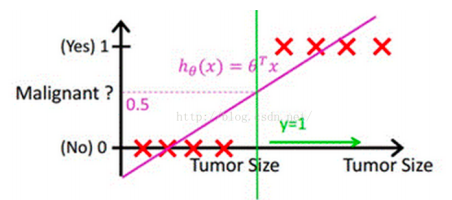但是这种情况是居于数据非常规则，没有什么异常噪声数据。如果有噪声则鲁棒性很差，就成下面的这种情况了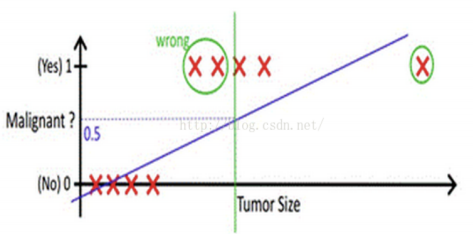可以看到无法找到一个合适的阈值来区分是不是恶性肿瘤。

逻辑回归详解

逻辑回归名称的由来

逻辑回归不是一个回归的算法，逻辑回归是一个分类的算法。那为什么逻辑回归不叫逻辑分类？因为逻辑回归算法是基于多元线性回归的算法。而正因为此，逻辑回归这个分类算法是线性的分类器。另外像一些基于决策树的一系列算法，基于神经网络的算法等那些是非线性的算法。SVM支持向量机的本质是线性的，但是也可以通过内部的核函数升维来变成非线性的算法。

逻辑回归和线性回归的决策函数

线性回归决策函数：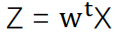将其通过sigmoid函数，获得逻辑回归的决策函数：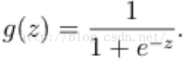说一下sigmoid

sigmoid函数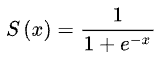Sigmoid 是一个可微的有界函数，在各点均有非负的导数。当 x→∞ 时，S(x)→1；当 x→−∞ 时，S(x)→0。常用于二元分类（Binary Classification）问题，以及神经网络的激活函数（Activation Function）（把线性的输入转换为非线性的输出）。

Logistic回归算法基于Sigmoid函数，或者说Sigmoid就是逻辑回归函数。

在逻辑回归中线性结果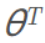通过sigmoid函数映射后的值被认为是属于y=1的概率，函数值域范围(0,1)。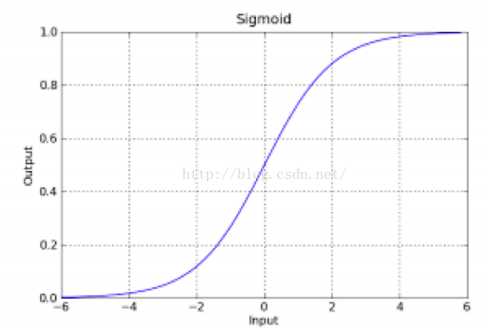因此逻辑回归表达式，也就是上边说的决策函数：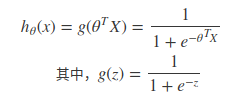sigmoid的导数形式：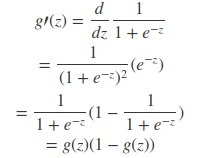逻辑回归决策函数结果中找分界线实现二分类

逻辑回归、线性回归属于监督学习范畴。

线性表达式，其值域为实数域，线性回归可以预测连续值，但是不能直接解决分类问题，我们需要根据预测的结果判定其属于正类还是负类(像上边例子中一样加一个阈值辅助就可以实现分类功能)

逻辑回归主要用来解决二分类问题（分类的本质是在空间中找到一个决策边界来完成分类的决策）。逻辑回归就是将线性回归的( − ∞ , + ∞ )结果，通过sigmoid函数映射到(0,1)之间的实数，这个实数代表概率，逻辑回归其实仅为在线性回归的基础上，套用了一个逻辑函数。线性函数的值越接近于正无穷大，概率值就越近1；反之，其值越接近于负无穷，概率值就越接近于0，这样的模型就是LR模型

我们知道二分类中  正例的概率+负例的概率 = 1。一个非常简单的试验是只有两个可能结果的试验，比如正面或反面，成功或失败，有缺陷或没有缺陷，病人康复或未康复。为方便起见，记这两个可能的结果为0和1。

分类器的本质就是要找到分界，目的最终要做二分类，因此逻辑回归的输出值要转换一下，最终输出0或者1。g(z)的值根据中间0.5分为二类，g(z)>0.5时我们看作概率为1，g(z)<0.5时看作概率为0。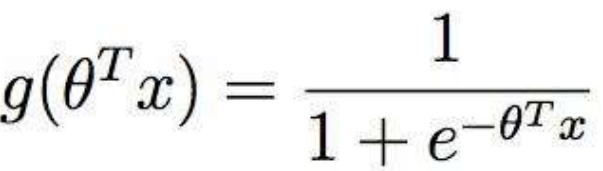当我们把0.5作为分界时，我们要找的就是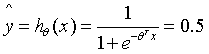的时候 θ 的解，即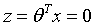时θ的解 ,在二维空间是一条直线，就是这条直线将样本分开达到分类的效果，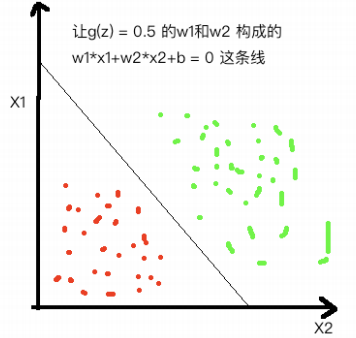分界线划分样本类别图

上图中直线左侧的样本 X ,通过计算对应的 g(z)是小于0.5的，反过来右边的是大于0.5的。

损失函数的推导

参数 θ 在很多情况下也会写作 w , x是样本，g(z)也可以写作g(w,x),这里我们依然用到最大似然估计思想，根据若干已知的X,y（训练集）找到一组W使得X作为已知条件下y发生的概率最大。逻辑回归中既然g(w,x)的输出含义为 P(y=1|w,x)，那么 P(y=0|w,x) = 1-g(w,x)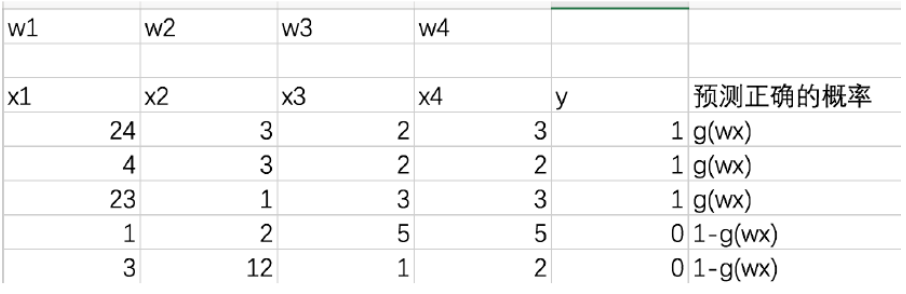只要根据训练集数据x和y，找到一组参数w, 让我们的g(w,x)函数在训练集上预测正确的概率最大，g(w,x)就是最好的解对于每一条数据预测正确的概率，也可以把下面公式看成是上面式子的合并形式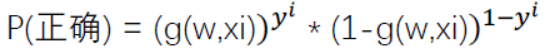换符号重写一下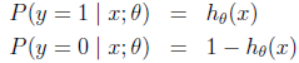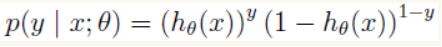我们假设训练样本相互独立，那么全部预测正确的概率= 每一条数据预测正确的概率相乘，因此似然函数表达式为：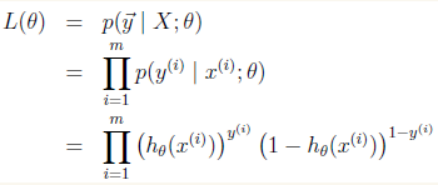转换对数似然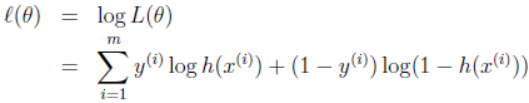这里还是最大化似然，加负号转换为最小似然,这就是我们的损失函数啦：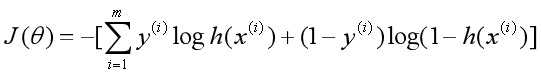总结，得到了逻辑回归的表达式，下一步跟线性回归类似，构建似然函数，然后最大似然估计，最终推导出θ的迭代更新表达式。另外通常我们一提到损失函数，往往是求最小，这样就可以用的是梯度下降求解，而不是梯度上升，所以最后由最大化似然函数加负号编程最小化似然函数。

梯度下降最优化模型

对参数theta θ求偏导，加上学习率的梯度下降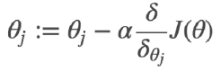利用上边sigmoid函数求导时的特性，对逻辑回归损失函数求导：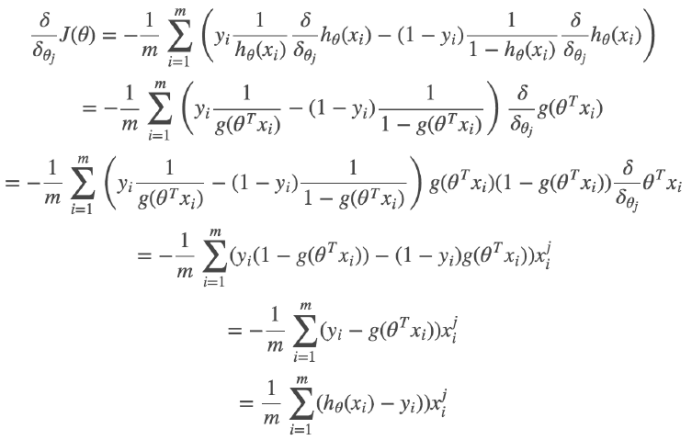毕竟都是从广义线性回归来的，我们发现导函数的形式和多元线性回归一样，对比详见：

机器学习算法之线性回归_RayChiu757374816的博客-CSDN博客

最终梯度下降：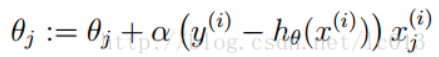最终问题转化为，在训练集上找到一组W使损失函数最小,这样就可以使得全部正确的概率最

逻辑回归的优化

分界线的截距必要性

我们回过头来再看一下上边的分界线划分样本类别图，我们可以看到分界线w1*x1 + w2*x2+b=0的截距是b ,我们可以看作是第一个w参数 w0,有这个截距和没有这个截距的区别如下图：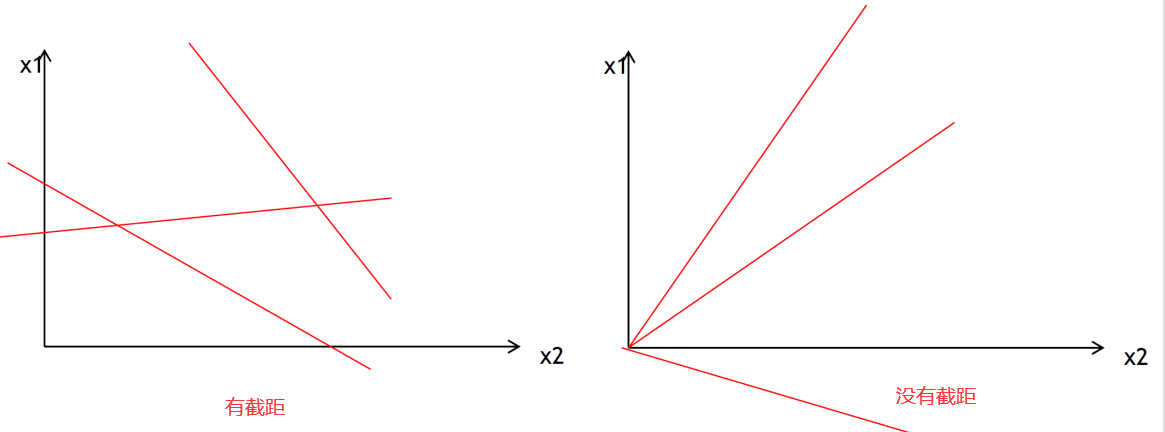可以看到有截距的分界线才有可能将数据做彻底的分类。

通过升维处理线性不可分的情况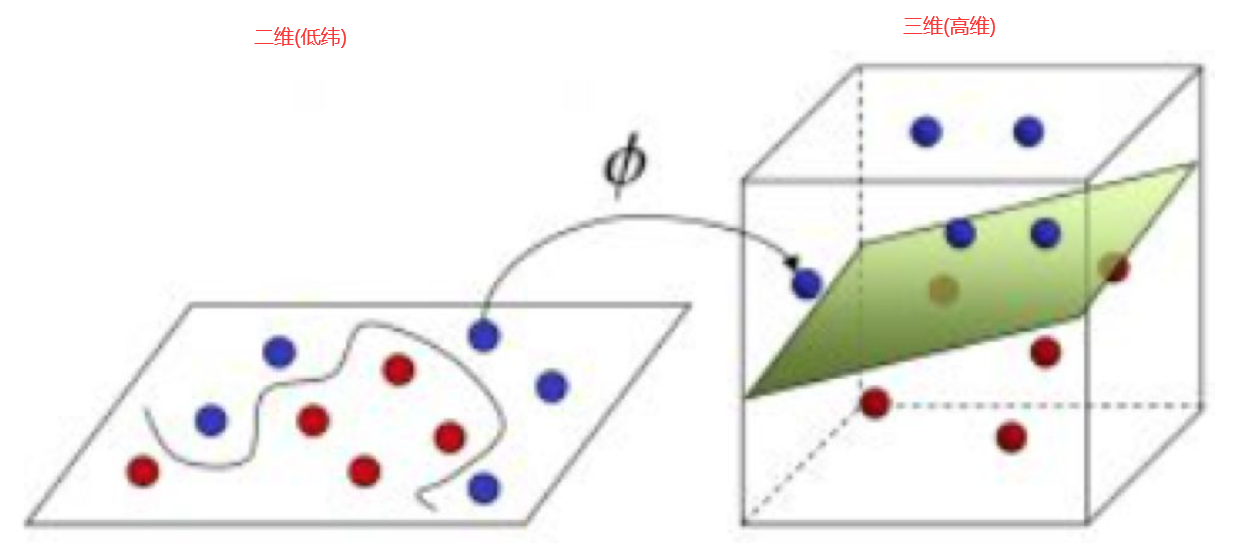对于线性不可分问题，可以使用升高维度的方式转换成线性可分问题。低维空间的非线性问题在高维空间往往会成为线性问题。

固定阈值0.5的调整

很多场景判别分类不是那么理想，比如样本不平衡，容错率的期望设定也不一样，如判断是否是癌症的场景，可以调整阈值牺牲一定错误率去规避一些不可接受的风险。

L1、L2正则化

正则化（Regularization）是机器学习中一种常用的技术，其主要目的是控制模型复杂度，减小过拟合。最基本的正则化方法是在原目标（代价）函数 中添加惩罚项，对复杂度高的模型进行“惩罚”。其数学表达形式为：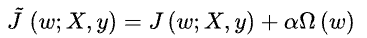上式中 X 、 y为训练样本和相应标签， W 为权重系数向量； J() 为目标函数，即为惩罚项，可理解为模型“规模”的某种度量；参数控制控制正则化强弱。不同的函数对权重的最优解有不同的偏好，因而会产生不同的正则化效果。最常用的函数有两种，即范数和范数，相应称之为正则化和正则化。此时有：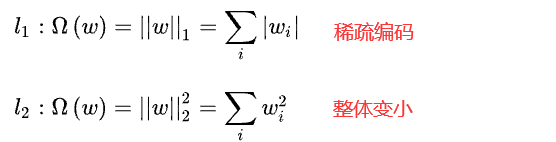目的是控制模型复杂度，减小过拟合

处理多分类问题

这个不用过多解释，多分类可以转化成多个二分类。

样本不均衡问题

处理方式有：前边说过的阈值调整、上采样 (upsampling) 或下采样 (downsampling)（又称过采样和欠采样）、人工创造新样本( 属性随机采样组成新的数据)、或者直接换决策树算法

【机器学习算法】逻辑回归调优 - cac2020 - 博客园

【如果对您有帮助，交个朋友给个一键三连吧，您的肯定是我博客高质量维护的动力！！！】

展开全文算法 机器学习
• 逻辑回归虽然名字里有回归(logistic regression)，实则它是个二分类算法。 从sigmoid函数引出 同多元线性回归一样，逻辑回归也具有它的函数表达式： hθ(x)=g(θTx)=11+e−θTx h_{\theta}(x)=g\left(\theta^{T} x\...

逻辑回归以及为什么叫逻辑回归

逻辑回归虽然名字里有回归(logistic regression)，实则它是个二分类算法。

从sigmoid函数引出

同多元线性回归一样，逻辑回归也具有它的函数表达式：
h θ ( x ) = g ( θ T x ) = 1 1 + e − θ T x h_{\theta}(x)=g\left(\theta^{T} x\right)=\frac{1}{1+e^{-\theta^{T} x}}
仔细观察这个表达式，会发现里面其实有我们见过的形式，右下角那个θᵀx不就是多元线性回归嘛。是的，没错，所以逻辑回归实则就是在多元线性回归的基础上，多嵌套了一个函数。我也不卖关子了，这个函数就是sigmoid函数，也被称作S型曲线：
σ ( x ) = 1 1 + e − x \sigma(x)=\frac{1}{1+e^{-x}}

x = np.linspace(-5,5,100)
sigmoid = 1/(1+np.exp(-x))
plt.plot(x,sigmoid)
plt.show()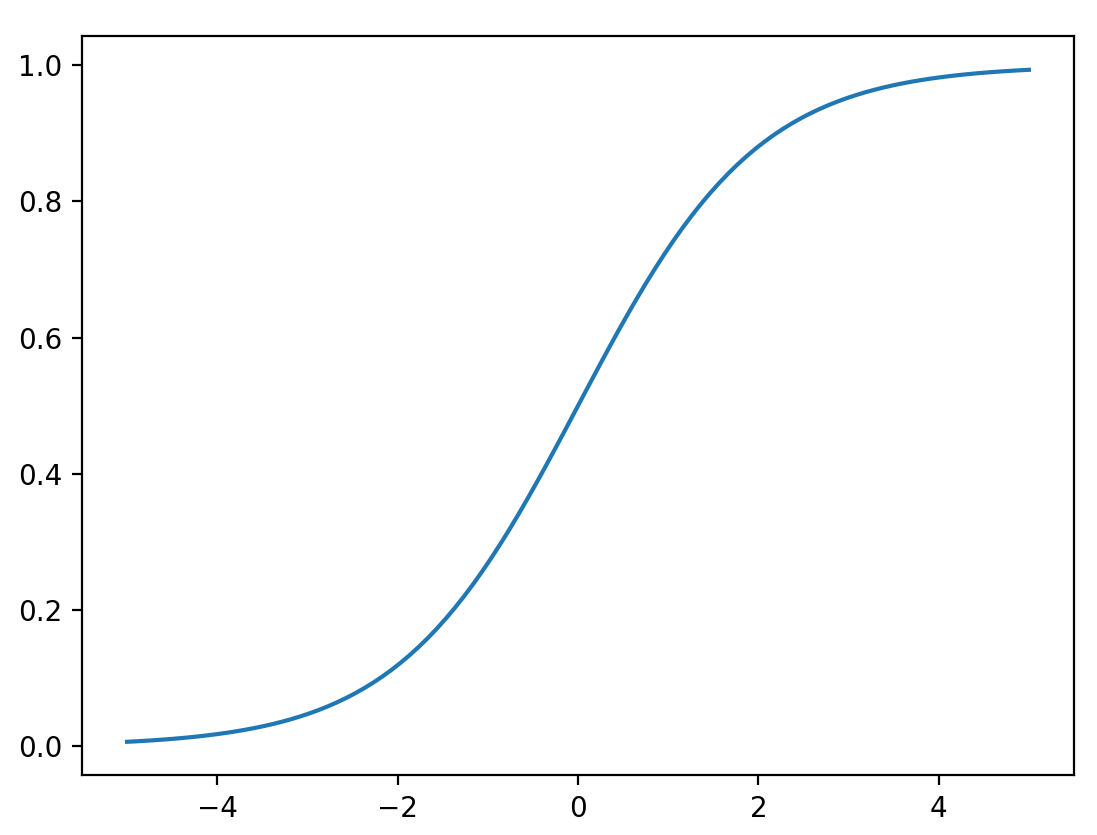可以看到sigmoid函数的值域为(0,1)。因此逻辑回归就是在多元线性回归基础上把结果缩放到 0 到 1 之间。 我们可以简单的先通过这个函数自我脑补一下逻辑回归为什么是一个分类算法：通过线性函数得到一个结果，这个结果越小于0，函数输出就越接近0，结果越大于0，函数输出就越接近1，而分类算法的输出一般情况下都会是input属于某个类别的概率大小，因此0到1之间的范围可以表示概率。中间的0.5则将结果划分为两个类别。所以我们可以简单的将sigmoid函数理解成是一个将输出转化为属于某个类别的概率的函数。

所以逻辑回归的大体思想就是，多元线性回归算法回归出一个分类超平面，预测样本通过代入这个超平面，如果是负的就说明在超平面下方，如果是正就说明是超平面上方。再通过sigmoid转化为概率，最后通过概率输出类别：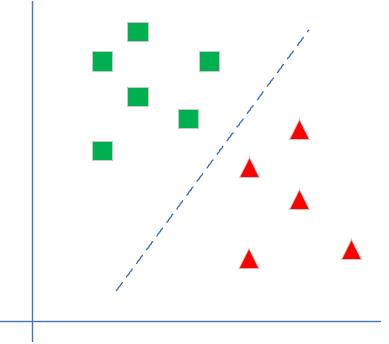那为什么是sigmoid，为什么sigmoid的输出就一定能表示概率，别的函数不行吗？

当然，任何算法一经给出，我们都需要知其然且知其所以然，即使不能窥其所有也要至少心里有个底。

逻辑回归的统计学模型：伯努利分布

我们知道，对于二分类算法，样本只有两个标签，要么是0要么是1，并且二分类有一个特点，那就是我们最终预测样本的概率，正例加上负例的概率总和为1。即每一次预测我们都可以这么表示：
P ⁡ ( X = 1 ) = p , P ⁡ ( X = 0 ) = 1 − p , 0 < p < 1 \operatorname{P}(X=1)=p, \operatorname{P}(X=0)=1-p, 0<p<1
这个公式看着是不是有点眼熟，这不就是伯努利分布(0-1分布)嘛！

对，因此，对于模型每一次预测的输出，我们都可以写成伯努利分布的形式：
f ( x ∣ p ) = { p x q 1 − x , x = 0 , 1 0 , x ≠ 0 , 1 f(x \mid p)= \begin{cases}p^{x} q^{1-x}, & x=0,1 \\ 0, & x \neq 0,1\end{cases}
伯努利分布有一个很典型的例子，就是抛硬币，只不过在抛硬币当中我们已经假设概率是对半分，但在逻辑回归中，概率是需要通过每一次模型预测给出的。

其实上面这一步，我们实则是为了给出P(y|x,p)服从伯努利分布的结论，这一结论实则是为了引出一个更强大的数学武器(接下来看不懂也无妨，我也只能在我理解的基础上尽量解释清楚。毕竟只是为了更好的知道sigmoid函数是怎么来的，觉得没太大意义的可以略过)

【这里再解释下，上述条件概率中的y就是模型预测的y，x就是输入x，y是x的函数，条件概率P的意思就是:在给定参数p(p也是待估计参数)以及输入的x的前提下，y发生的概率。而这里的结论是逻辑回归的二分类任务的输出是服从伯努利分布的】

线性回归模型的通式：广义线性模型

首先通俗解释下什么是广义线性模型，比如说家喻户晓的多元线性回归，它的形式是y = wTx+b,更一般的，考虑到某可微函数g(.),令：
g ( y ) = w T x + b g(y)=w^Tx+b

y = g − 1 ( w T x + b ) y = g^{-1}(w^Tx+b)
对于逻辑回归而言，其g(.)满足：
g ( y ) = l n ( y 1 − y ) g(y) = ln(\frac{y}{1-y})
现在，考虑一个分类或回归问题，我们就是想预测某个随机变量 y，y 是某些特征 x 的函数。广义线性模式遵循如下三个假设：

1.p(y|x;θ)服从指数族分布，θ就是模型中待学习的变量

2.我们的目的是为了预测T(y)在条件x下的期望，并且一般情况下T(y)=y，即y_hat=E(y|x)

3.参数η和输入x是线性相关的，即η=θᵀx

指数族分布

这里首先解释下什么是指数族分布，指数族分布有：高斯分布、二项分布、伯努利分布、多项分布、泊松分布、指数分布、beta 分布、拉普拉斯分布、gamma 分布。

对于回归来说，如果因变量 y 服从某个指数族分布，那么我们就可以用广义线性回归来建模。比如说如果 y 是服从伯努利分布，我们可以使用逻辑回归（也是一种广义线性模型），或者如果y服从高斯分布，那可以用线性回归。

这里直接给出指数族分布的通用形式：
p ( y ; η ) = b ( y ) exp ⁡ ( η T T ( y ) − a ( η ) ) p(y ; \eta)=b(y) \exp \left(\eta^{T} T(y)-a(\eta)\right)
η 是 自然参数（natural parameter）。

T(y) 是充分统计量 （sufficient statistic） ，一般情况下就是 y。

b(·)和a(·)都是函数。a(η) 是 对数部分函数（log partition function），这部分确保 Y 的分布 p(y:η) 计算的结 果加起来（连续函数是积分）等于 1。

我们上面其实已经给出了结论，那就是伯努利分布就是指数族分布的一种，不过这里再证明下也无妨：

伯努利分布(φ就是概率)：
p ( y ; ϕ ) = ϕ y ( 1 − ϕ ) 1 − y p(y ; \phi)=\phi^{y}(1-\phi)^{1-y}
可以把上式右边改写成指数分布族的形式(以e为底)：
p ( y ; ϕ ) = exp ⁡ ( y ln ⁡ ϕ + ( 1 − y ) ln ⁡ ( 1 − ϕ ) ) = exp ⁡ ( ( ln ⁡ ( ϕ 1 − ϕ ) ) y + ln ⁡ ( 1 − ϕ ) ) \begin{aligned} &p(y ; \phi)=\exp (y \ln \phi+(1-y) \ln (1-\phi)) \\ &=\exp \left(\left(\ln \left(\frac{\phi}{1-\phi}\right)\right) y+\ln (1-\phi)\right) \end{aligned}
这里我们就可以一一对照一下，
b ( y ) = 1 η T = ln ⁡ ( p 1 − p ) = θ T x T ( y ) = y a ( η ) = − ln ⁡ ( 1 − ϕ ) \begin{aligned} & b(y)=1 \\ &\eta^T=\ln \left(\frac{p}{1-p}\right)=\theta^{T} x\\ &T(y)=y\\ &a(\eta)=-\ln (1-\phi) \end{aligned}
这里η是个数值，所以转不转置都没关系。

由此可知：
η T = θ T x = ln ⁡ ( p 1 − p ) e θ T x = p 1 − p p = e θ T x − e θ T x ⋅ p = e θ T x 1 + e θ T x = 1 1 + e − θ T x \begin{aligned} &\eta^T=\theta^{T} x=\ln \left(\frac{p}{1-p}\right)\\ &e^{\theta^{T} x} =\frac{p}{1-p} \\ &p =e^{\theta^{T} x}-e^{\theta^{T} x} \cdot p \\ &=\frac{e^{\theta^{T} x}}{1+e^{\theta^{T} x}} \\ &=\frac{1}{1+e^{-\theta^{T} x}} \end{aligned}
啊哈,最下面那个形式不就是sigmoid吗，顺便一提，这里的ln[p/(1-p)]在数学上还有个名字，叫做对数几率。然后我又顺带一查，惊喜的发现，原来对数几率回归的英文名就是logistic regression！

这下子，虽然对上面的推导过程还有些一知半解，但是至少明白了逻辑回归中的回归到底在回归些什么。

我们知道，多元线性回归的表达式是在拟合那条直线。其实逻辑回归就是用着多元线性回归的表达式，在拟合对数几率，只不过这个拟合的过程只是作为一个中间步骤，最后拟合出的对数几率又通过sigmoid函数转化为二分类的概率，所以本质上逻辑回归还是一个披着分类的大衣的回归算法。

既然都提到了广义线性回归，那我们这里再提一下多元线性回归也无妨，毕竟多元线性回归是假设样本的误差服从高斯分布，高斯分布也是指数族分布的一种。

因为多元线性回归假设样本同方差，所以我们假设方差为1不影响：
p ( y ; μ ) = 1 2 π exp ⁡ ( − 1 2 ( y − μ ) 2 ) p(y ; \mu)=\frac{1}{\sqrt{2 \pi}} \exp \left(-\frac{1}{2}(y-\mu)^{2}\right)
写成指数族分布的形式就是;
= 1 2 π exp ⁡ ( − 1 2 y 2 ) ⋅ exp ⁡ ( μ y − 1 2 μ 2 ) =\frac{1}{\sqrt{2 \pi}} \exp \left(-\frac{1}{2} y^{2}\right) \cdot \exp \left(\mu y-\frac{1}{2} \mu^{2}\right)
由广义线性模型的假设，我们是为了预测y在条件x下的期望，这里期望其实就是μ。并且μ=η=θᵀx

所以y_hat=θᵀx。这也就是多元线性回归的表达式。

展开全文机器学习 逻辑回归 算法
• 1、逻辑回归 2、公式推导 也就是说如果x区域正无穷的时候，概率值也就越接近1，对数几率也就接近1。 对数几率优点： 直接对分类的概率建模，无需实现假设数据分布，从而避免了假设分布不准确带来的问题...

1、逻辑回归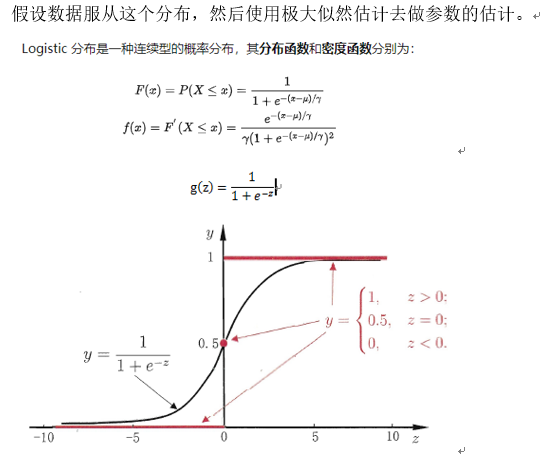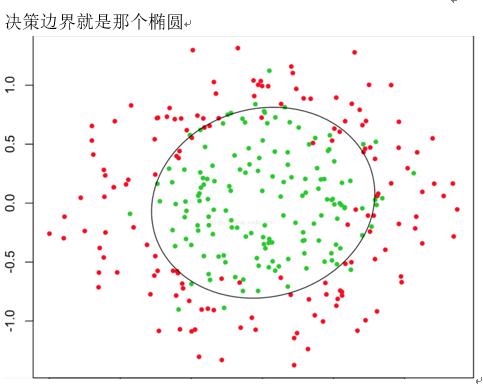2、公式推导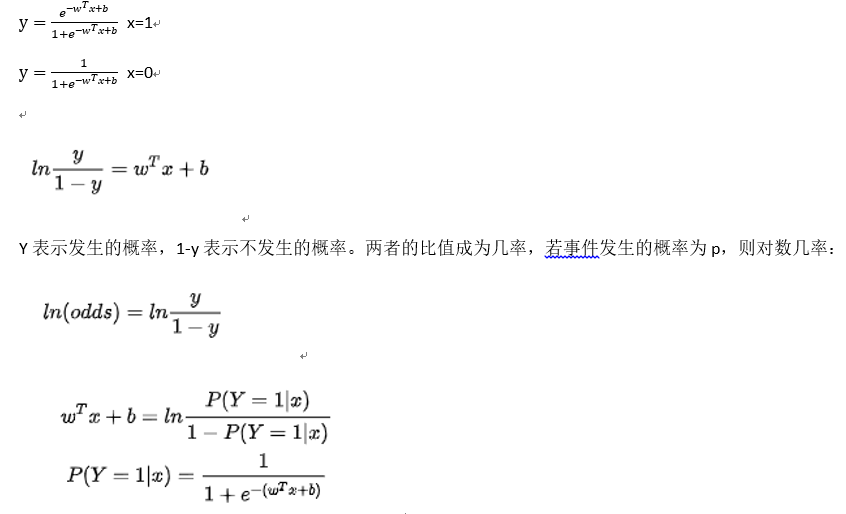也就是说如果x区域正无穷的时候，概率值也就越接近1，对数几率也就接近1。

对数几率优点：

• 直接对分类的概率建模，无需实现假设数据分布，从而避免了假设分布不准确带来的问题（区别于生成式模型（朴素贝叶斯）
• 不仅可以预测类别还可以预测概率，对于决策任务很有用。
• 对数几率函数是任意阶可导的凸函数，有许多函数值优化算法都可以求出最优解。

3、损失函数

既然使用极大似然估计法来求解，必须明确似然函数。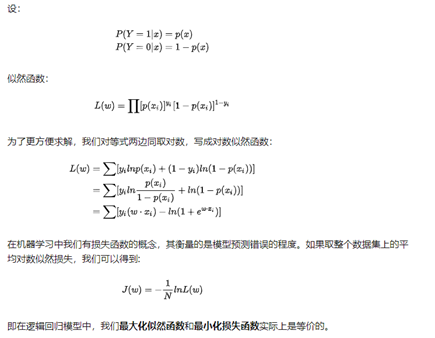4、求解

逻辑回归的损失函数是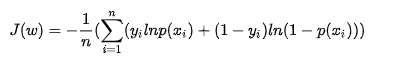• 随机梯度下降算法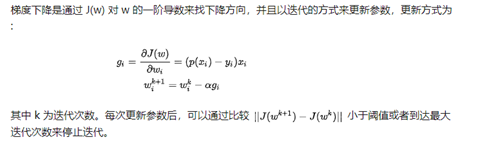• 牛顿法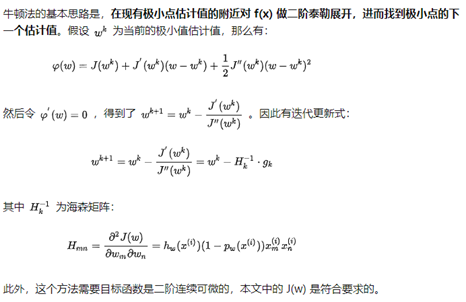5、正则化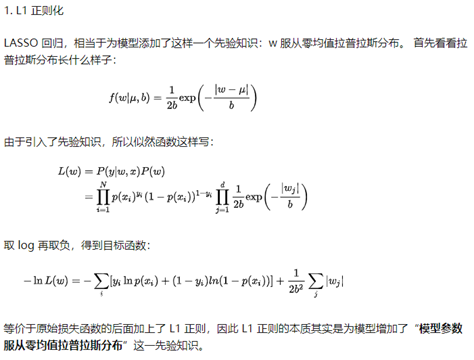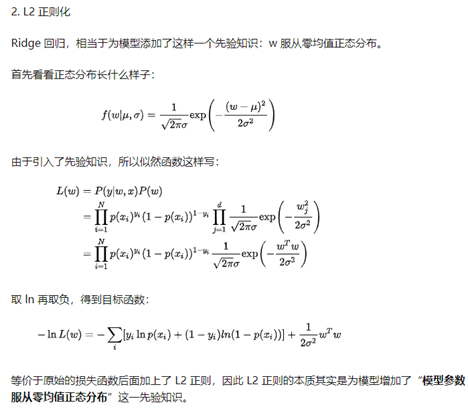6、并行化

无论是梯度下降还是牛顿法都需要计算梯度，逻辑回归的并行化主要就是对目标函数梯度计算的并行化。目标函数的梯度向量计算中需要进行向量间的点乘和相加，可以很容易将每个迭代过程拆分成相互独立的计算步骤，由不同的节点进行独立计算，然后归并计算结果。

7、和SVM对比

• 相同点

• 都是分类算法，本质都是在寻找最佳分类超平面
• 都是监督学习算法
• 都是判别式模型，不关心数据如何生成，只关心数据之间的差别
• 都可以增加正则项
• 不同点

• LR是一个统计的方法，SVM是一个几何的方法
• SVM只考虑support vector
• 损失函数不同：LR是交叉熵，SVM是HingerLoss
• LR是参数模型；SVM是非参数模型

8、优缺点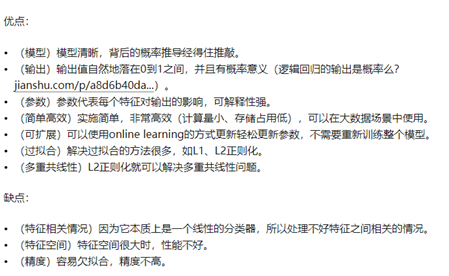9、参考

展开全文逻辑回归 机器学习 算法
• 机器学习——逻辑回归算法代码实现前言一、逻辑回归是什么？二、代码实现1.数据说明2.逻辑回归代码 前言 最近准备开始学习机器学习，后续将对学习内容进行记录，该文主要针对逻辑回归代码实现进行记录！同时也准备...

前言

最近准备开始学习机器学习，后续将对学习内容进行记录，该文主要针对逻辑回归代码实现进行记录！

一、逻辑回归是什么？

逻辑回归概念篇可看博主之前的文章，传送门

二、代码实现

1.数据说明

你想根据两次考试的结果来决定每个申请人的录取机会。你有以前的申请人的历史数据，你可以用它作为逻辑回归的训练集。对于每一个培训例子，你有两个考试的申请人的分数和录取决定。为了做到这一点，我们将建立一个分类模型，根据考试成绩估计入学概率。
数据源下载：数据下载 提取码: 5mf2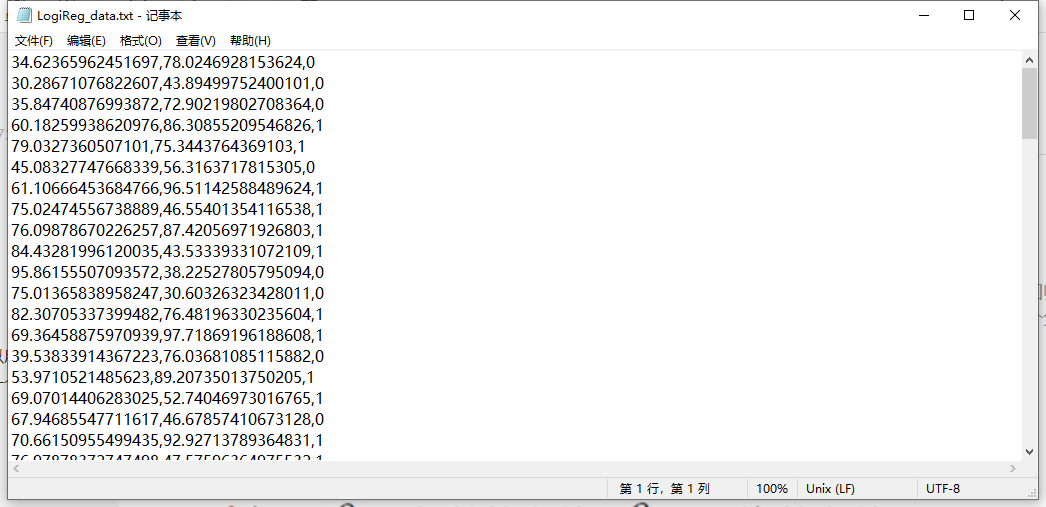数据导入

#三大件
import numpy as np
import pandas as pd
import matplotlib.pyplot as plt
%matplotlib inline
# 可以在Ipython编译器里直接使用，功能是可以内嵌绘图，并且可以省略掉plt.show()这一步
import os
path = 'data' + os.sep + 'LogiReg_data.txt' #改成数据源路径

输出为：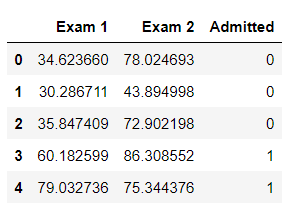将数据用散点图绘制出来

positive = pdData[pdData['Admitted'] == 1] # returns the subset of rows such Admitted = 1, i.e. the set of *positive* examples
negative = pdData[pdData['Admitted'] == 0] # returns the subset of rows such Admitted = 0, i.e. the set of *negative* examples

fig, ax = plt.subplots(figsize=(10,5))
ax.scatter(positive['Exam 1'], positive['Exam 2'], s=30, c='b', marker='o', label='Admitted')
ax.scatter(negative['Exam 1'], negative['Exam 2'], s=30, c='r', marker='x', label='Not Admitted')
ax.legend()
ax.set_xlabel('Exam 1 Score')
ax.set_ylabel('Exam 2 Score')

输出为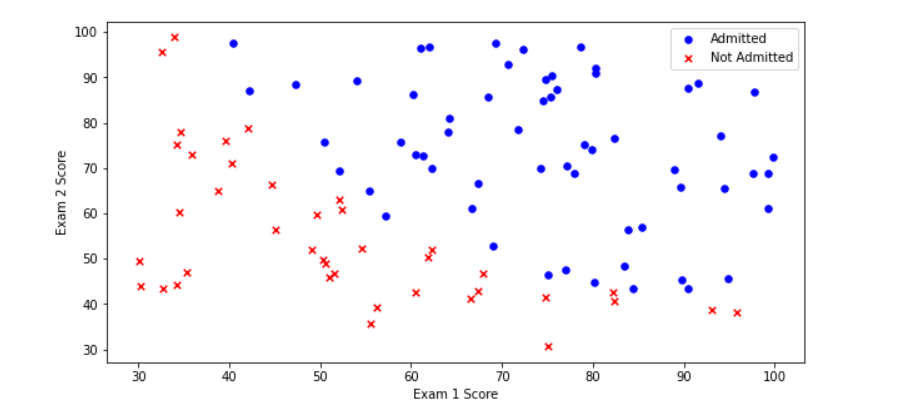2.逻辑回归代码

目标：建立分类器（求解出三个参数 θ 0 θ 1 θ 2 \theta_0\theta_1\theta_2 ）设定阈值，根据阈值判断录取结果(一般设置为0.5)
待完成模块：
·sigmoid : 映射到概率的函数
·model : 返回预测结果值
·cost : 根据参数计算损失
·descent : 进行参数更新
·accuracy: 计算精度

具体代码说明：
①sigmoid函数

def sigmoid(z):
return 1 / (1 + np.exp(-z))

②model函数

def model(X, theta):
return sigmoid(np.dot(X, theta.T))

③增加一列取值为1的特征

if 'Ones' not in pdData.columns:
pdData.insert(0, 'Ones', 1) # in a try / except structure so as not to return an error if the block si executed several times

④将pandas转换为ndarray，获取数据总列数

orig_data = pdData.values # convert the Pandas representation of the data to an array useful for further computations
cols = orig_data.shape

⑤提取特征数据X，结果数据y，定义 θ \theta

X = orig_data[:,0:cols-1]
y = orig_data[:,cols-1:cols]
theta = np.zeros([1, 3])

⑥损失函数
J ( θ ) = 1 n ∑ i = 1 n [ ( − y l o g ( h θ ( x ) ) ) − ( 1 − y ) l o g ( 1 − h θ ( x ) ) ] J(\theta)=\frac{1}{n}\sum_{i=1}^n[(-ylog(h_\theta(x)))-(1-y)log(1-h_\theta(x))]

def cost(X, y, theta):
left = np.multiply(-y, np.log(model(X, theta)))
right = np.multiply(1 - y, np.log(1 - model(X, theta)))
return np.sum( left-right) / (len(X)) #n取决于传递进来的X样本的个数，根据不同的梯度下降方法对应不同的n

⑦计算梯度方向

error = (model(X, theta)-y ).ravel() #拉成一个一维数组
for j in range(len(theta.ravel())): #for each parmeter
term = np.multiply(error, X[:,j]) #相当于乘了每个样本的第j个特征
grad[0, j] = np.sum(term) / len(X) #n取决于传递进来的X样本的个数，根据不同的梯度下降方法对应不同的n

⑧比较3中不同梯度下降方法

STOP_ITER = 0#根据迭代次数停止
STOP_COST = 1#根据损失停止

def stopCriterion(type, value, threshold):
#设定三种不同的停止策略
if type == STOP_ITER:        return value > threshold
elif type == STOP_COST:      return abs(value[-1]-value[-2]) < threshold
elif type == STOP_GRAD:      return np.linalg.norm(value) < threshold

import numpy.random
#洗牌
def shuffleData(data):
np.random.shuffle(data)
cols = data.shape
X = data[:, 0:cols-1]
y = data[:, cols-1:]
return X, y

import time
#batchsize=1表示随机梯度下降，batchsize=100表示批量梯度下降，为其他表示小批量
def descent(data, theta, batchSize, stopType, thresh, alpha):
#梯度下降求解

init_time = time.time()
i = 0 # 迭代次数
k = 0 # batch
X, y = shuffleData(data)
costs = [cost(X, y, theta)] # 损失值

while True:
k += batchSize #取batch数量个数据
if k >= n:
k = 0
X, y = shuffleData(data) #重新洗牌
theta = theta - alpha*grad # 参数更新
costs.append(cost(X, y, theta)) # 计算新的损失
i += 1

if stopType == STOP_ITER:       value = i
elif stopType == STOP_COST:     value = costs
if stopCriterion(stopType, value, thresh): break

return theta, i-1, costs, grad, time.time() - init_time

def runExpe(data, theta, batchSize, stopType, thresh, alpha):
#import pdb; pdb.set_trace();
theta, iter, costs, grad, dur = descent(data, theta, batchSize, stopType, thresh, alpha)
name = "Original" if (data[:,1]>2).sum() > 1 else "Scaled"
name += " data - learning rate: {} - ".format(alpha)
elif batchSize==1:  strDescType = "Stochastic"
else: strDescType = "Mini-batch ({})".format(batchSize)
name += strDescType + " descent - Stop: "
if stopType == STOP_ITER: strStop = "{} iterations".format(thresh)
elif stopType == STOP_COST: strStop = "costs change < {}".format(thresh)
else: strStop = "gradient norm < {}".format(thresh)
name += strStop
print ("***{}\nTheta: {} - Iter: {} - Last cost: {:03.2f} - Duration: {:03.2f}s".format(
name, theta, iter, costs[-1], dur))

fig, ax = plt.subplots(figsize=(12,4))
ax.plot(np.arange(len(costs)), costs, 'r')
ax.set_xlabel('Iterations')
ax.set_ylabel('Cost')
ax.set_title(name.upper() + ' - Error vs. Iteration')
return theta

对结果进行绘图分析：

#选择的梯度下降方法是基于所有样本的，迭代50000次，每一次步长为0.000001
n=100
runExpe(orig_data, theta, n, STOP_ITER, thresh=5000, alpha=0.000001)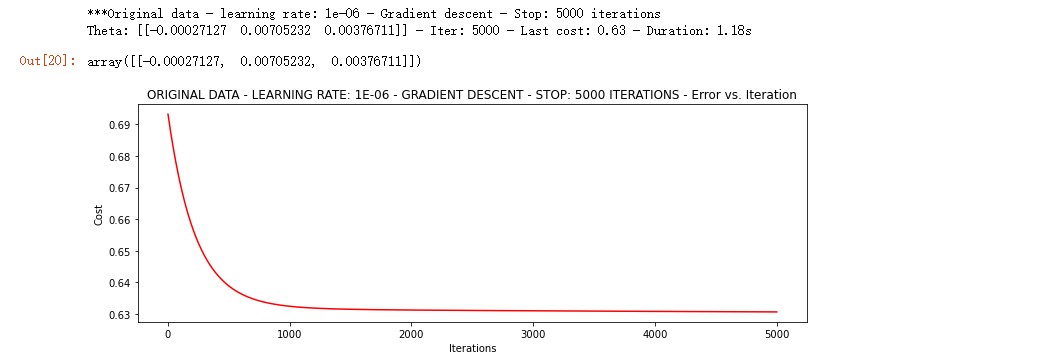runExpe(orig_data, theta, n, STOP_COST, thresh=0.000001, alpha=0.001)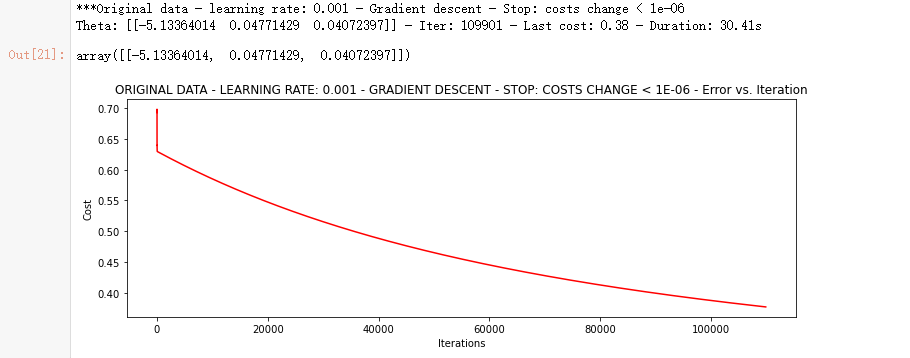runExpe(orig_data, theta, n, STOP_GRAD, thresh=0.05, alpha=0.001)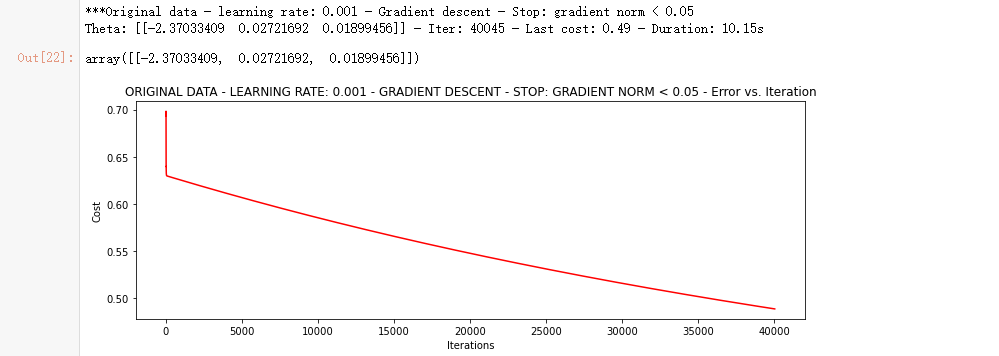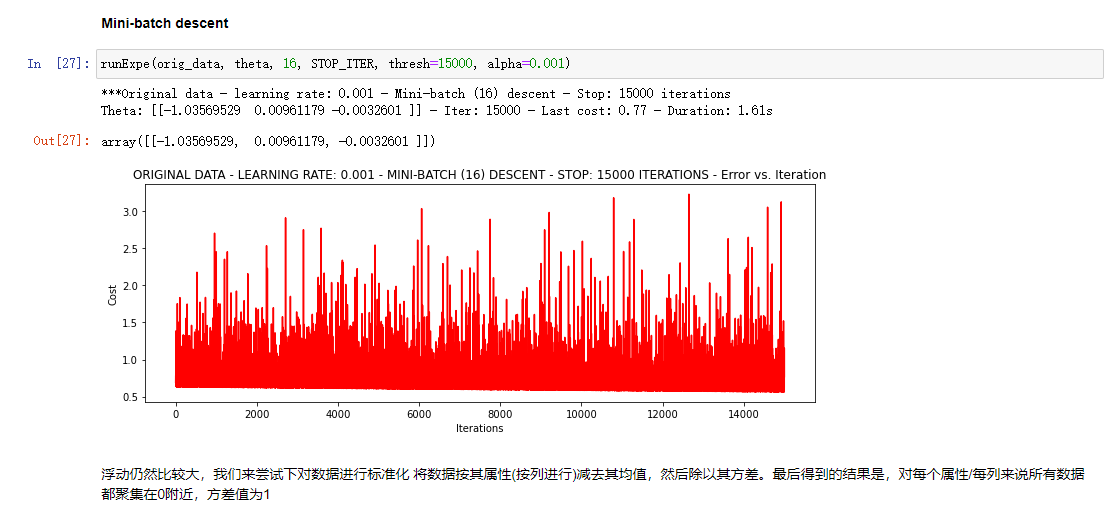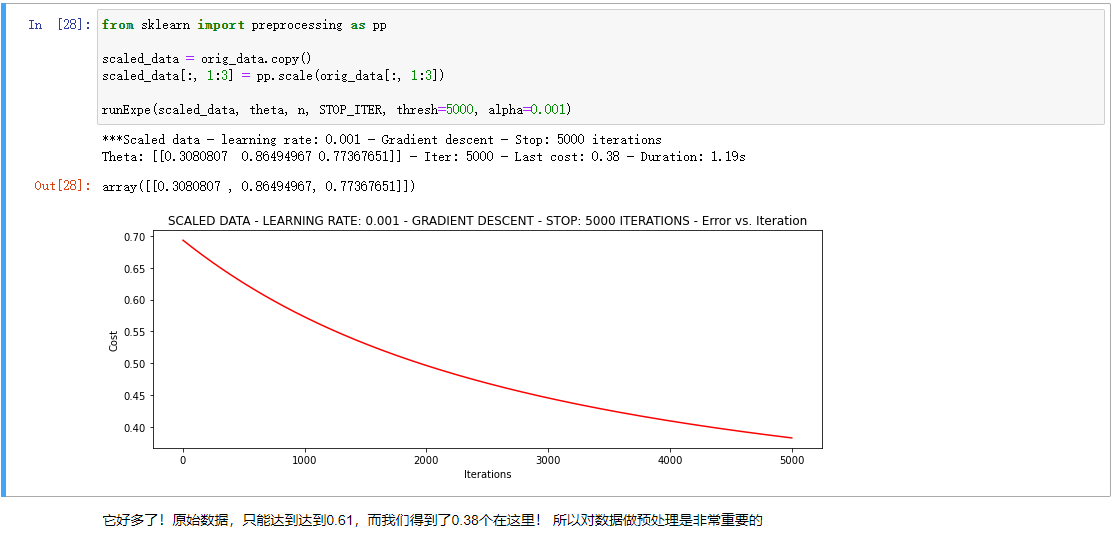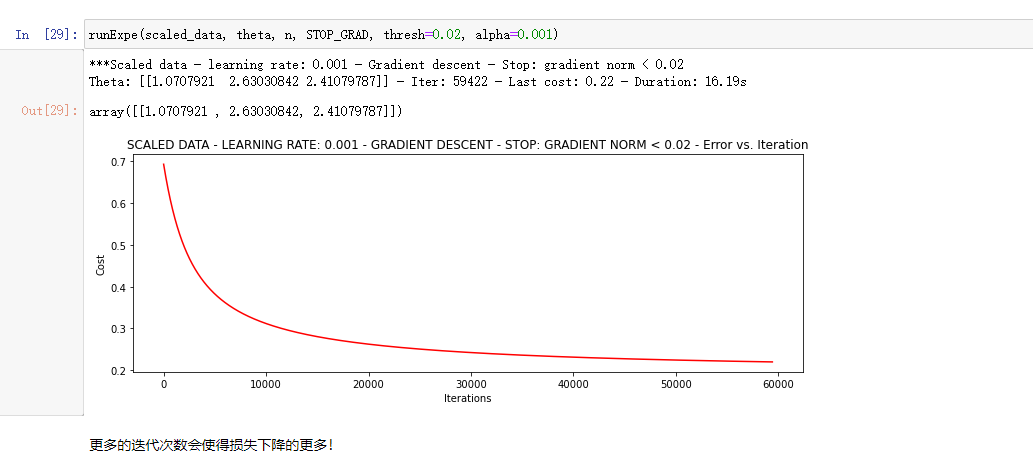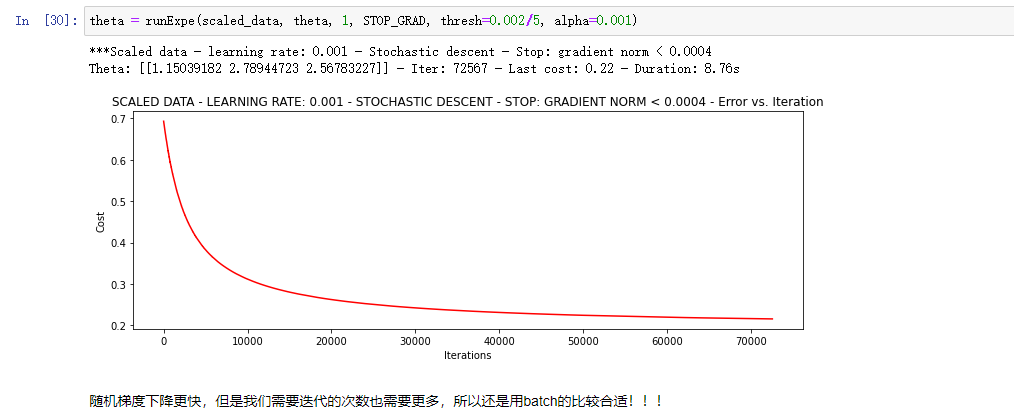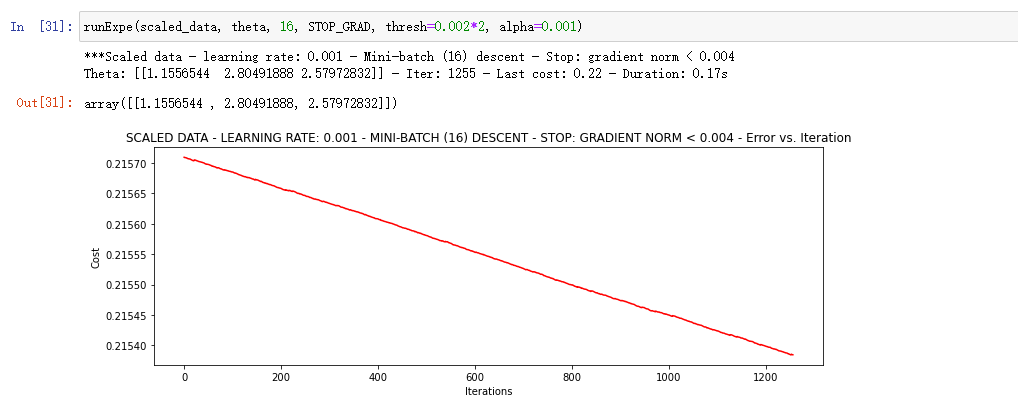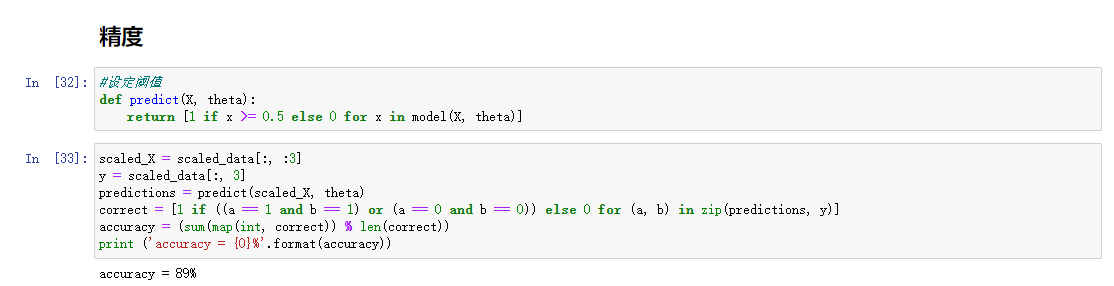展开全文机器学习 python 逻辑回归
• 环境spark-1.6python3.5一、有无截距对于逻辑回归分类，就是找到z那条直线，不通过原点有截距的直线与通过原点的直线相比，有截距更能将数据分类的彻底。packagecom.bjsxt.lrimportorg.apache.spark.mllib....
• 逻辑回归 原理简介： Logistic回归虽然名字里带“回归”，但是它实际上是一种分类方法，主要用于两分类问题（即输出只有两种，分别代表两个类别），所以利用了Logistic函数（或称为Sigmoid函数），函数形式为： 其...机器学习
•机器学习 sklearn 逻辑回归
• part, test_size = 0.2, random_state = 2020) # random_state为随机数种子 # 从sklearn中导入逻辑回归模型 from sklearn.linear_model import LogisticRegression # 定义 逻辑回归模型 clf = LogisticRegression...机器学习
• 1 逻辑回归的介绍和应用 1.1 逻辑回归的介绍 逻辑回归（Logistic regression，简称LR）虽然其中带有"回归"两个字，但逻辑回归其实是一个分类模型，并且广泛应用于各个领域之中。虽然现在深度学习相对于这些传统方法...可视化 机器学习 python
• 逻辑回归同步更新在个人网站：http://www.wangpengcufe.com/machinelearning/pythonml-pythonml6/一、概述1.1、概念是一种名为“回归”的线性分类器，是由线性回归变化而来的，一种广泛使用于分类问题中的广义回归...
• 逻辑回归算法 最近参加一个阿里巴巴举办的机器学习训练营，正好在此记录下自己的学习笔记。 提到逻辑回归算法，不知道你是不是把它当做了一个回归算法，我是第一印象把它当做了回归算法，而且我觉得这个不就是线性...机器学习 线性代数
• 在公众号「python风控模型」里回复关键字：学习资料，免费领取。 python风控模型 持牌照金融公司模型专家，教学主页 https://ke.qq.com/teacher/231469242?tuin=dcbf0ba机器学习 逻辑回归 人工智能
• 文章目录系列文章逻辑回归1 逻辑回归介绍1.1 逻辑回归原理输入激活函数1.2 损失以及优化2 逻辑回归API介绍3 案例：肿瘤良性恶性预测4 分类模型的评估4.1 分类评估方法混淆矩阵准确率精确率召回率F1-score4.2 分类...机器学习 python 逻辑回归算法
• 机器学习算法（一）：基于逻辑回归的分类预测 而对于逻辑回归而且，最为突出的两点就是其模型简单和模型的可解释性强。 逻辑回归模型的优劣势: 优点：实现简单，易于理解和实现；计算代价不高，速度很快，存储资源...逻辑回归 机器学习 算法
• 逻辑回归：logistic回归是一种广义的线性回归，通过构造回归函数，利用机器学习来实现分类或者预测。 线性模型：通过特征的线性组合预测的函数。其一般向量格式为： 线性模型具有较好的可解释性，w表示不同特征...逻辑回归
• 导入数据包，实现逻辑回归时，可以使用梯度下降算法，也可以直接使用高级优化算法（共轭梯度法），更加简便。使用高级优化算法可以自动选择学习率。 import numpy as np import pandas as pd import matplotlib....python 机器学习 深度学习 算法 逻辑回归
• 逻辑回归：Logistic Regression，Logit Regression，是一种分类算法，常用于处理二分类，用来表示某件事情发生的可能性。任务是尽可能地拟合决策边界。 应用：银行信用卡欺诈可能性（是欺诈消费、不是欺诈消费）、...机器学习 算法 逻辑回归
• 逻辑回归（LR） 我的理解是，逻辑回归就是以改进的线性回归的方法求分类，改进的内容就是引入了非线性函数，最常用的就是sigmoid函数。 如下图就是sigmoid函数，横轴z = 0时，纵轴g(z) = 0.5，z趋向于负无穷时，g(z)...算法 机器学习
• 1、线性回归 分类：一元线性回归、多元线性回归 优缺点分析： 优点：模型简单、运算量小，即使数据量很大，仍然可以快速得到结果 模型的参数就是特征的权重，具有很好的解释性 缺点：对异常值敏感 当数据...机器学习
• 逻辑回归是监督学习，主要解决二分类问题。 逻辑回归虽然有回归字样，但是它是一种被用来解决分类的模型，为什么叫逻辑回归是因为它是利用回归的思想去解决了分类的问题。 逻辑回归和线性回归都是一种广义的线性...机器学习 逻辑回归 sklearn python
• 逻辑回归内部也使用梯度下降算法 数据集资源 本文基于鸢尾花 数据集实现 数据集：数据集网盘下载 提取码：p2v9 数据集简单处理 import numpy as np import pandas as pd data = pd.read_csv(r"dataset/iris.arff....python 机器学习 逻辑回归
• 这里先给出阿里云机器学习训练营地址：阿里云机器学习训练营，可以将其代码下载进行学习或者参加最后一个任务的比赛。 1 逻辑回归的介绍和应用 1.1 逻辑回归的介绍 逻辑回归（Logistic regression，简称LR）虽然...机器学习
• 然后将数据集分为训练集和测试集，由于数据比较少，所以用20%的比例分离数据集，再用logistic回归算法进行预测，分别用degree = 1 或者 2 或者等等用来模拟数据，选出最适合的模型，再绘制学习曲线，对模型进行...机器学习 回归
• 本系列为机器学习算法的总结和归纳，目的为了清晰阐述算法原理，同时附带上手代码实例，便于理解。 目录 k近邻（KNN） 决策树 线性回归 逻辑斯蒂回归 朴素贝叶斯 支持向量机（SVM） 组合算法（Ensemble Method） K-...机器学习 线性回归
• 本篇博文主要介绍了机器学习里面的逻辑回归，并给出了相关的计算过程，最后使用主流的机器学习库sklearn来实现逻辑回归机器学习(手推公式版)系列持续更新中...人工智能 机器学习 python 逻辑回归 算法
• 机器学习算法专题（蓄力计划）】十四、机器学习中逻辑回归算法 python 机器学习 深度学习 逻辑回归
• 机器学习实验二——逻辑回归 实验报告 组员：张继伟 谢正宇 樊佳婷 孙晶铭 刘怡聪 题目 假设你是某大学招生主管，你想根据两次考试的结果决定每个申请者的录取机会。现有以往申请者的历 史数据，可以此作为训练集...
• 现在我们将按照上面相同的步骤来训练各种机器学习算法逻辑回归（Logistic Regression） model = LogisticRegression() model.fit(train_X,train_y) prediction=model.predict(test_X) print('The accuracy of the...逻辑回归 机器学习 支持向量机
• 总的来说，机器学习的问题可以分为两大类：分类和回归，不管是分类还是回归，其本质是一样的，都是对输入做出预测，并且都是监督学习。说白了，就是根据特征，分析输入的内容，判断它的类别，或者预测其值。 在分类...算法 机器学习 sklearn
• 机器学习算法（一）: 基于逻辑回归的分类预测 1 逻辑回归的介绍和应用 1.1 逻辑回归的介绍 逻辑回归（Logistic regression，简称LR）虽然其中带有"回归"两个字，但逻辑回归其实是一个分类模型，并且广泛应用于各个...机器学习 python 可视化...